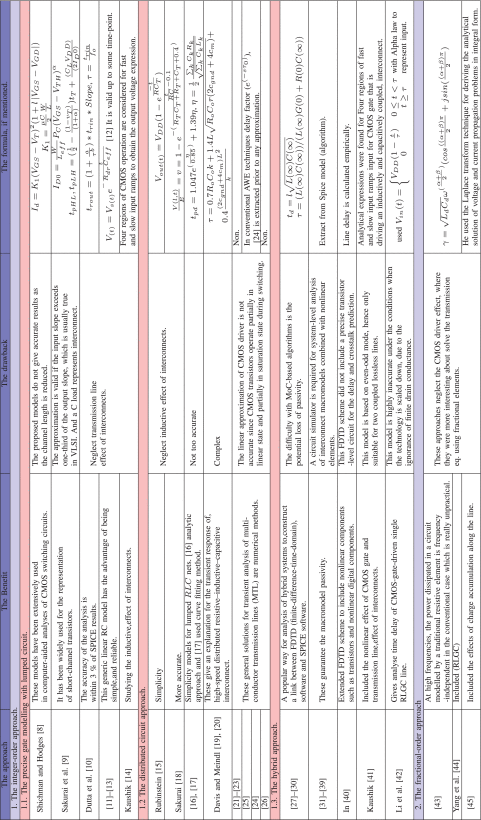Journal

# Advance Interconnect Circuit Modeling Design Using Fractional-Order Elements

By
Al-Daloo M.
Soltan A.
Yakovlev A.

Nowadays, the interconnect circuits' conduct plays a crucial role in determining the performance of the CMOS systems, especially those related to nano-scale technology. Modeling the effect of such an influential component has been widely studied from many perspectives. In this article, we propose a new general formula for RLC interconnect circuit model in CMOS technology using the fractional-order elements approach. The study is based on approximating an infinite transfer function of the CMOS circuit with a noninteger distributed RLC load to a finite number of poles. It is accurate due to the effect of adding fractional-order variables and since these variables are utilized for tuning the model to match the design regardless of its complexity. As such, delay calculations employing our analytical model are within 0.4 absolute error of COMSOL-computed delay across a range of interconnect lengths. Furthermore, the effect of the interconnect conductivity G has been taken into account tacitly although the model included the resistance R, inductance L, and capacitance C of the interconnect. A number of analyses were set up at different levels of the design to evaluate the effectiveness. First, demonstrating the significant effects of generalizing parameters was gained by studying the fractional-order impedance and propagation constant of the transmission line for a range of frequencies. Second, using MATLAB we assessed the potential of the proposed approximated model besides the exact one, which shows similarity in the fundamental features of the system, such as stability and resonance. Third, the proposed approach showed that with a very small tuning reach 0.01 of the generalizing parameters can achieve up to 15% improvement in the model accuracy. © 1982-2012 IEEE.